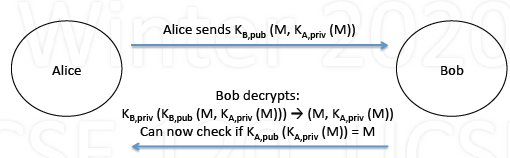0%

## Basic

1. What is Computer Security?

• How to protect computer systems

• System contents: data, software, hardware
• System operation: performance, reliability
• System service: what the user sees and expects
• From various threats

• Theft
• Damage
• Disruption
2. Aspects of Security

• Confidentiality

• keeping a secret secret; for authorized eyes only
• Integrity

• maintaining accuracy; only authorized changes
• Authenticity

• Is it really who/what it claims to be?
• Availablity

• access to info/resources you need, when needed
3. Security Threats

• Interception

• eavesdropping
• Interruption

• destroying, denial of service
• Modification

• tampering with data or programs
• Fabrication

• new data/programs, replaying message

## User Authentication

1. Password

• Passwords are most common method

• User and computer know secret
• User proves knowledge of secret
• Computer checks
• Encrypted passwords

• Computer stores only encrypted passwords
• User provides password
• Computer encrypts, checks
• Problem with passwords

• Assume 100 possible characters for passwords

| # chars| # passwords | 100G/s machine | 100T/s machine |
| :—-: | :—-: | :—-: | :—-: |
| 6 | $100^6$ | 10 sec | 10 msec |
| 7 | $100^7$ | 17 min | 10 msec |
| 8 | $100^8$ | 1.2 days | 1.7min |
| 9 | $100^9$ | 116 days | 2.8 hr|

• But most characters are uncommon, hard to remember

• Using dictionary words (~250,000): only 2.5 usec!
2. Challenge/Response Protocol

• Challenge/response, algorithm passwords

• User and system know secret algorithm
• System challenge user’s knowledge, user responds
• Example: say secret algorithm is $f(x) = x^2$

• System challenges user: sends system 9
• User computes $f(x) = 9$, sends system 9
• System concludes user must know secret algorithm
• Next time, system can provide different challenge
• Secret is never sent, only challenge/response

## Threats

1. Trojan Horse

• Greeks invaded Troy in hollow wooden horse
• Program that contains hidden malicious code

• User thinks program does something useful
• In actuality, it (also) does something harmful
• Program runs as process in user’s domain

• Can do harm to user’s environment
• Can do harm under that user’s name
2. Trap Door

• Secret access point in program
• Designer develops program for someone else
• Once loaded in system, designer can access
• Consider if trap door is added by compiler

• Compiler adds trap doors to programs
• Designer of compiler can then access
• Can’t tell from program source code
• Even if new compiler written, must be compiled!
3. Virus

• Code attached to legitimate program
• When program runs, the virus runs

• causes damage
• spreads, attaching itself to other programs
• Disinfectants

• Check that programs look normal (modified)
• Check for known virus patterns in programs
4. Internet Worm

• Worm: program that copies itself over network by email, finger, rsh attack
5. Denial of Service

• Preventing others from using system

• by using lots of resources
• by bombarding with network requests or traffic
• Example

• Repeatly request TCP connection
• Don’t answer responses; system times them out
• Eventually, no TCP ports left available

## Approach to clear (prevent) threats

### Intrusion Detection

1. Intrusion Detection

• Detecting if there is an intruder, or an attack
• Signature-based

• Look for specific patterns of attack behavior
• Example: repeated login attempts
• Anomaly-based

• Look for unusual behavior
• Example: unusual command/system call patterns
• Solution: create audit trail (log), then analyze it

### Crytography

1. Basics

• Encoding messages to

• limit who can view the original message
• determine who sent a message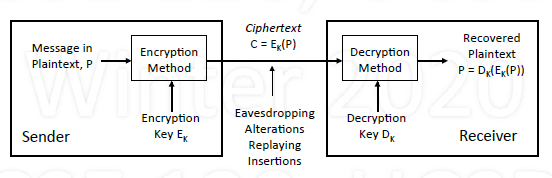2. Secret Key Encryption

• Secret key (symmetric)

• Same key K is used to encrypt and decrypt
• Sender encrypts $E_k(P)$, Receiver decrypts $D_k(E_k(P))$
• DES: Data Encryption Standard (1997)

• Weak due to 56-bit keys
• AES: Advanced Encryption Standard (2001)

• 128, 192, 256-bit keys
3. Public Key Encryption

• Public key (asymetric)

• Different keys to encrpyt and decrypt
• Each user has two keys: one public, one private
• If A wants to send to B

• A encrypts using B’s public key
• B decrypts using its private key
• RSA (Rivest, Shamir and Adelman)

4. Public key vs. Secret key

• Secret key

• Operates fast
• Difficult to distribute keys
• Public key

• Time-consuming operation (generating random/prime number, see example below)
• Conveninet for key distribution
• Example: Alice chats with Bob

• Bob authenticates Alice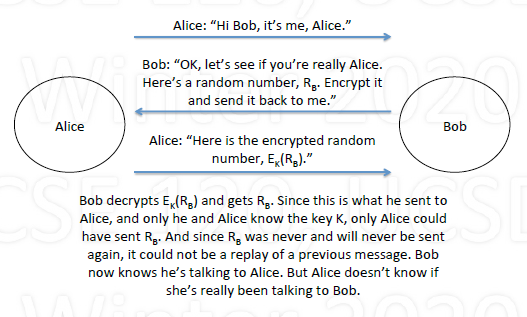• Alice authenticates Bob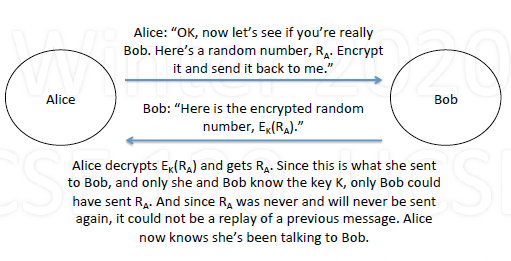• Authentication using public key

• Alice: sends $K_{B,pub}(A, R_A)$ to Bob (uses Bob’s public key)
• Bob:

• Decrypts: $K{B,priv}(K{B,pub}(A, R_A))\rightarrow (A, R_A)$
• Encrypts and sends $K_{A,pub}(R_A, R_B, K)$ to Alice
• Alice:

• Decrypts: $K{A,priv}(K{A,pub}(R_A, R_B, K))\rightarrow$ “It’s Bob”
• Encrypts and sends $K(R_B)$ to Bob
• Bob: Decrypts $K(K(R_B)) = R_B \rightarrow$ “it’s Alice”

• whole process: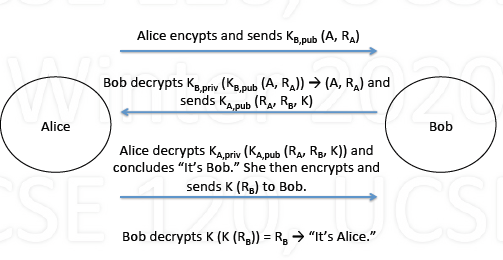5. Digitial Signatures

• If Alice wants to digitially sign message to Bob

• Encrypt M using $K{A,priv}$ and send $K{A,priv}(M)$ to Bob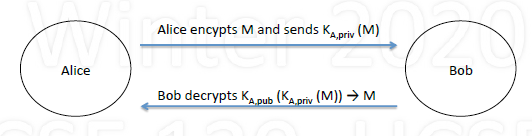• When Bob receives, decrypts using $K_{A, pub}$

• can decrypt only if from Alice
• To sign and keep private

• Alice sends $K{B,pub}(M, K{A, pirv}(M))$ to Bob
• Only Bob can decryptL $K{B,priv}(K{B,pub}(M, K_{A,priv}(M))$
• Decrypts using $K_{A,pub}$ proving Alice signed it